Next: Bibliography

The Hamiltonian constraint in the loop representation of quantum gravity

John C. Baez

Department of Mathematics, University of California
Riverside, California 92521
USA

email: baez@math.ucr.edu

August 27, 1996

In July and the first part of August there was a workshop on Mathematical Problems of Quantum Gravity at the Erwin Schrödinger Institute in Vienna, run by Peter Aichelburg and Abhay Ashtekar. One of the goals of this workshop was to gather together people working on the loop representation of quantum gravity and have them tackle some of the big open problems in this subject. For some time now, the most important outstanding problem has been to formulate the Wheeler-DeWitt equation in a rigorous way by making the Hamiltonian constraint into a well-defined operator. Just before the workshop began, Thomas Thiemann put four papers aimed at solving this problem onto the preprint archive gr-qc . This led to quite a bit of excitement as the workshop participants began working through the details. A personal account of the workshop as a whole can be found on my website ; here I wish only to give an introduction to Thiemann's work. In the interests of brevity I will not attempt to credit the many people whose work I allude to.

An interesting feature of Thiemann's approach is that while it uses the whole battery of new techniques developed in the loop representation of quantum gravity, in some respects it returns to earlier ideas from geometrodynamics. Recall that in geometrodynamics á la Wheeler and DeWitt, the basic canonically conjugate variables were the 3-metric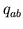and extrinsic curvature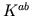. The idea was to quantize these, making them into operators acting on wavefunctions on the space of 3-metrics, and then to quantize the Hamiltonian and diffeomorphism constraints and seek wavefunctions annihilated by these quantized constraints. However, this program soon became regarded as dauntingly difficult for various reasons, one being the non-polynomial nature of the Hamiltonian constraint: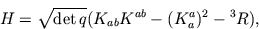whereis the scalar curvature of the 3-metric. It is often difficult to quantize non-polynomial expressions in the canonically conjugate variables and their derivatives. The factor of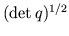is not even an entire function of the 3-metric!

In the 1980's Ashtekar found a new formulation of general relativity in which the canonically conjugate variables are a densitized complex triad field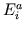and a chiral spin connection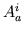. When all the constraints are satisfied, these are related to the original geometrodynamical variables by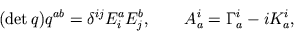where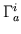is built from the Levi-Civita connection of the 3-metric and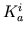is built from the extrinsic curvature. In terms of these new variables the Hamiltonian constraint appears polynomial in form, reviving hopes for canonical quantum gravity.

Actually, in this formulation one works with the densitized Hamiltonian constraint, given by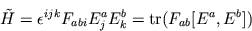where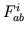is the curvature of, and the trace and commutator are interpreted by thinking of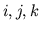as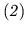indices. Clearly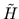is a polynomial in,, and their derivatives. However, it is related to the original Hamiltonian constraint by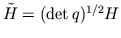, so in a sense the original problem has been displaced rather than addressed. It took a while, but it was eventually seen that many of the problems with quantizingcan be traced to this fact (or technically speaking, the fact that it has density weight 2).

A more immediately evident problem was that becauseis complex-valued, the corresponding 3-metric is also complex-valued unless one imposes extra reality conditions'. The reality conditions are easy to deal with in the Riemannian theory, where the signature of spacetime is taken to be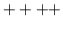. There one can handle them by working with a real densitized triad fieldand an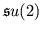connection given not by the above formula but by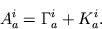In the physically important Lorentzian theory, however, no such easy remedy is available.

Despite these problems, the enthusiasm generated by the new variables led to a burst of work on canonical quantum gravity. Many new ideas were developed, most prominently the loop representation. In the Riemannian theory, this allows one to rigorously construct a Hilbert space of wavefunctions on the space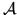ofconnections on space. The idea is to work with graphs embedded in space, and for each such graph to define a Hilbert space of wavefunctions depending only on the holonomies of the connection along the edges of the graph. Concretely, if the graph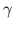has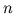edges, the holonomies along its are summarized by a point in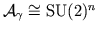, and the Hilbert space we get is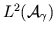, defined using Haar measure on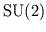. If the graphis contained in a larger graph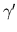then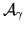is contained in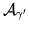and one has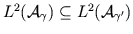. We can thus form the union of all these Hilbert spaces and complete it to obtain the desired Hilbert space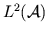.

One can show thathas a basis of spin networks', given by graphs with edges labeled by representations of-- i.e., spins -- and vertices labeled by vectors in the tensor product of the representations labeling the incident edges. One can also rigorously quantize geometrically interesting observables such as the total volume of space, obtaining operators on. The matrix elements of these operators can be explicitly computed in the spin network basis.

Thiemann's approach applies this machinery developed for the Riemannian theory to Lorentzian gravity by exploiting the interplay between the Riemannian and Lorentzian theories. He takes as his canonically conjugate variables anconnectionand a real densitized triad field, and takes as his Hilbert spaceas defined above. This automatically deals with the reality conditions, as in the Riemannian case. Then he writes the Lorentzian Hamiltonian constraint in terms of these variables, and quantizes it to obtain a densely defined operator on-- modulo some subtleties we discuss below. Interestingly, it is crucial to his approach that he quantizes the Hamiltonian constraint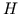rather than the densitized Hamiltonian constraint. This avoids the regularization problems that plagued attempts to quantize.

He writes the Lorentzian Hamiltonian constraintin terms ofandin a clever way, as follows. First he notes that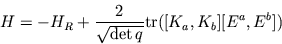where the commutators and trace are taken in, and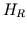is the Riemannian Hamiltonian constraint, given by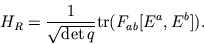Then he notes that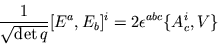where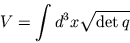is the total volume of space (which is assumed compact). This observation lets him get rid of the terrifying factors of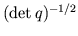. Similarly, he notes that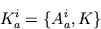where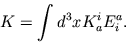Thus he obtains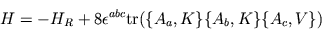where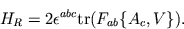Finally, he eliminates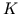from the formula forusing the formula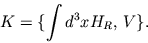If we use the standard trick of replacing Poisson brackets by commutators, these formulas reduce the problem of quantizingto the problem of quantizing,, and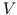. As noted, the volume has already been successfully quantized, and the resulting volume operator' is known quite explicitly. This leaves the connection and curvature.

Now, a fundamental fact about the loop representation -- at least as currently formulated -- is that the connection and curvature do not correspond to well-defined operators on, even if one smears them with test functions in the usual way. Instead, one has operators corresponding to the holonomy along paths in space. The holonomy along an open path can be used to define a kind of substitute for, and the holonomy around an open loop to define a substitute for. One cannot, however, take the limit as the path or loop shrinks to zero length. Thus the best one can do when quantizing a polynomial inandis to choose some paths or loops and use the substitutes built from holonomies. This eliminates problems associated with multiplying operator-valued distributions, but it introduces another kind of ambiguity: dependence on the arbitrary choice of path or loop.

So, ironically, while the factors ofin the Hamiltonian constraint are essential in Thiemann's approach, the polynomial expressions inandintroduce problematic ambiguities! Accepting but carefully minimizing this ambiguity, Thiemann obtains for any lapse function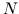a large family of different versions of the smeared Hamiltonian constraint operator. The ambiguity is such that two different versions acting on a spin network give spin networks differing only by a diffeomorphism of space. Mathematically speaking we may describe this as follows. Let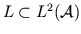be the space of finite linear combinations of spin networks, and let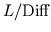be the space of finite linear combinations of spin networks modulo diffeomorphisms. Then Thiemann obtains, for any choice of lapse function, a Hamiltonian constraint operator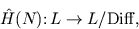independent of the arbitrary choices he needed in his construction.

Since these operators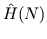do not map a space to itself we cannot ask whether they satisfy the naively expected commutation relations, the Dirac algebra'. However, this should come as no surprise, since the Dirac algebra also involves other operator-valued distributions that are ill-defined in the loop representation, such as. Thiemann does check as far as possible that the consequences one would expect from the Dirac algebra really do hold. Thus if one is troubled by how arbitrary choices of paths and loops prevent one from achieving a representation of the Dirac algebra, one is really troubled by the assumption, built into the loop representation, that,, andare not well-defined operator-valued distributions. Ultimately, the validity of this assumption can only be known through its implications for physics. The great virtue of Thiemann's work is that it brings us closer to figuring out these implications.Next: Bibliography
John Baez 2000-11-21